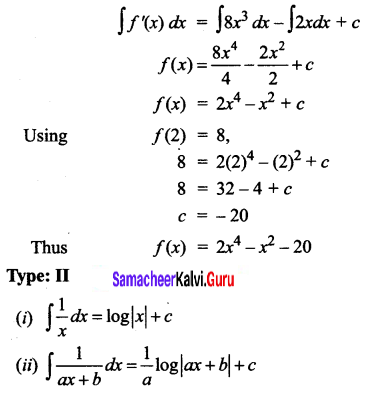# Samacheer Kalvi 12th Business Maths Solutions Chapter 2 Integral Calculus I Ex 2.1

Students can download 12th Business Maths Chapter 2 Integral Calculus I Ex 2.1 Questions and Answers, Samacheer Kalvi 12th Business Maths Book Solutions Guide Pdf helps you to revise the complete Tamilnadu State Board New Syllabus and score more marks in your examinations.

## Tamilnadu Samacheer Kalvi 12th Business Maths Solutions Chapter 2 Integral Calculus I Ex 2.1

Integrate the following with respect to x.

Question 1.
$$\sqrt{3 x+5}$$
Solution: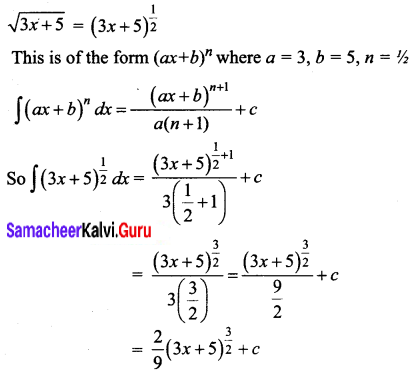Question 2.
$$\left(9 x^{2}-\frac{4}{x^{2}}\right)^{2}$$
Solution: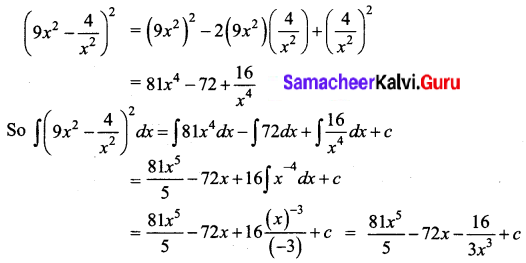Question 3.
(3 + x)(2 – 5x)
Solution: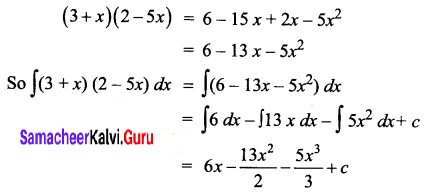Question 4.
√x(x3 – 2x + 3)
Solution: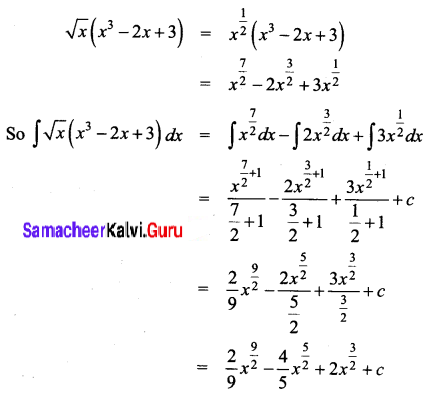Question 5.
$$\frac{8 x+13}{\sqrt{4 x+7}}$$
Solution: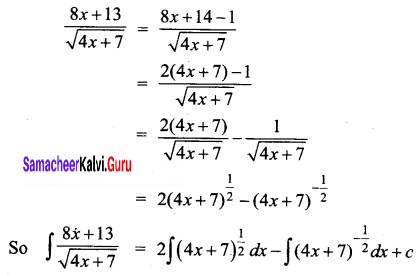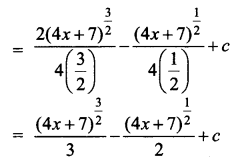Question 6.
$$\frac{1}{\sqrt{x+1}+\sqrt{x-1}}$$
Solution: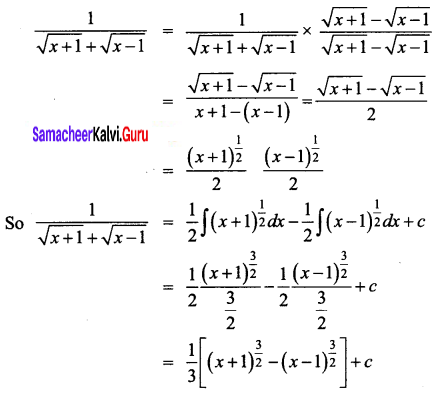Question 7.
If f'(x) = x + b, f(1) = 5 and f(2) = 13, then find f(x)
Solution:
f'(x) = x + b
Integrating both sides of the equations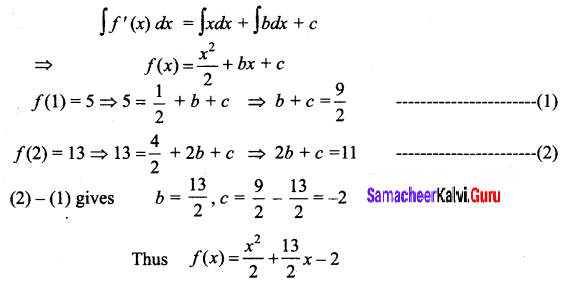Question 8.
If f(x) = 8x3 – 2x and f(2) = 8, then find f(x)
Solution:
f'(x) = 8x3 – 2x
Integrating both sides of the equation,